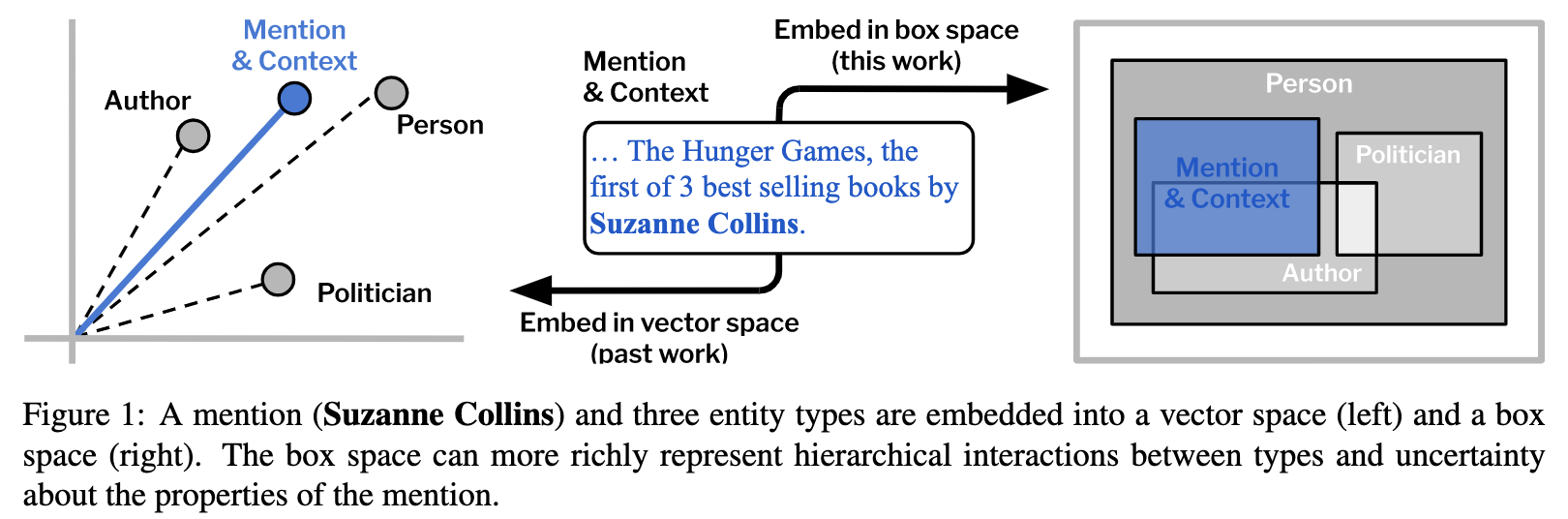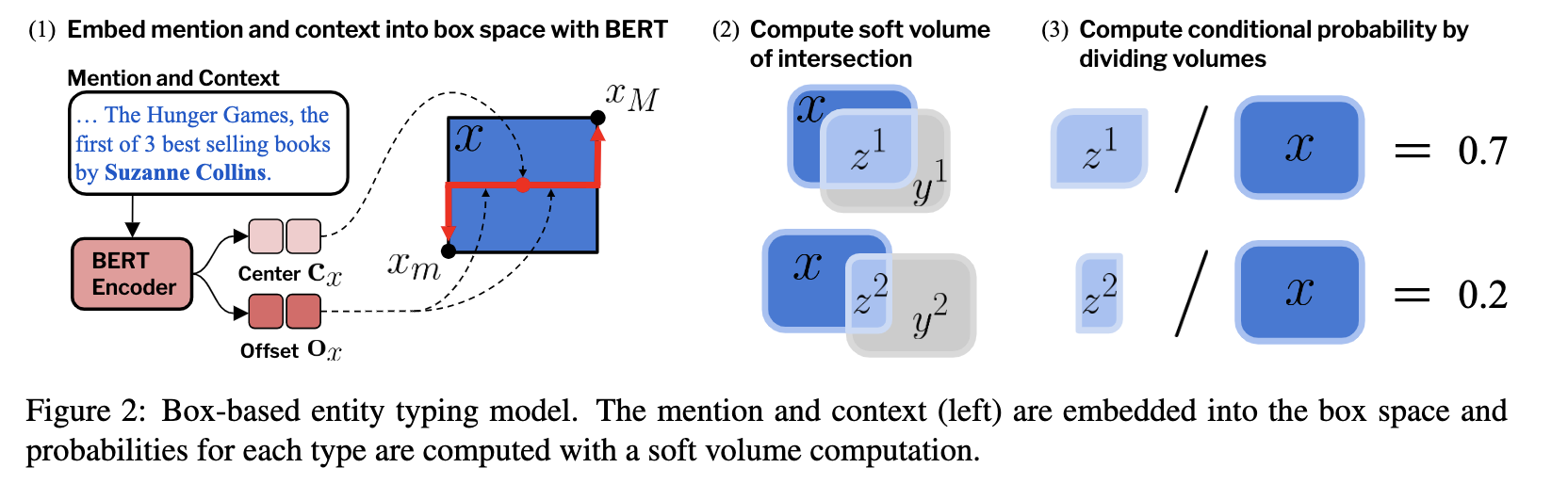# Modeling Fine-Grained Entity Types with Box Embeddings

Modeling Fine-Grained Entity Types with Box Embeddings

## Modeling Fine-Grained Entity Types with Box Embeddings

2. 本文使用了Box Embeddings理论来解决细粒度实体链接任务，作者认为传统的欧式空间无法建模复杂的类别关系（如层次、包含等），而Box Embeddings则有比较良好的几何性质，作者给出了一个很形象的例子：如左图所示，欧式空间不同实体类别无法建模层次结构，而有图中Box Embeddings可以通过包含、交叉来蕴含类别间的子类关系和相关关系，这是本文的一个出发点。1. 每个Box Embedding由两个参数控制，分别是左下顶点$x_{m}$和右上顶点$x_{M}$，计算得到这两个点即可确定一个box。（从几何上很好理解）
2. 给定两个box (x, y)，如何计算似然概率：
$$P(y \mid x)=\frac{\operatorname{Vol}(x \cap y)}{\operatorname{Vol}(x)}$$
其中Vol可理解为容积，是一个非常重要的概念，按照下式计算（i代表第i个维度）：
$$\operatorname{Vol}(x)=\prod_{i}\left(x_{M, i}-x_{m, i}\right) \\ \operatorname{Vol}(x \cap y)=\prod_{i} \max \left(\min \left(x_{M, i}, y_{M, i}\right)-\max \left(x_{m, i}, y_{m, i}\right), 0\right)$$
实际计算时会使用下式：
$$\operatorname{Vol}(x) \approx \prod_{i} \text { softplus }\left(\frac{x_{M, i}-x_{m, i}}{\beta}-2 \gamma\right)$$
而$z = x \cap y$则由下式计算：
\begin{aligned} &z_{m}^{k}=\beta \ln \left(e^{\frac{x_{m}}{\beta}}+e^{\frac{y_{m}^{m}}{\beta}}\right) \\ &z_{M}^{k}=-\beta \ln \left(e^{-\frac{x_{M}}{\beta}}+e^{-\frac{y_{M}^{k}}{\beta}}\right) \end{aligned}

$$p_{\theta}\left(t^{k} \mid m, s\right)=\frac{\operatorname{Vol}\left(z^{k}\right)}{\operatorname{Vol}(x)}=\frac{\operatorname{Vol}\left(x \cap y^{k}\right)}{\operatorname{Vol}(x)}$$

1. 使用BERT获取mention and context编码，再通过一个MLP得到隐层$\overline{\mathbf{h}} \in \mathbb{R}^{2 d}$，其中前d维是Box Embedding的center $\mathbf{C}_{x} \in \mathbb{R}^{d}$，后d维是Box Embedding的offset $\mathbf{o}_{x} \in \mathbb{R}^{d}$。对于每个实体类别类似，只不过center和offset是随机初始化得到。
2. 然后根据center和offset计算box embedding：$$x_{m}=\sigma\left(\mathbf{c}_{x}-\operatorname{SoFTPLUS}\left(\mathbf{o}_{x}\right)\right) \\ x_{M}=\sigma\left(\mathbf{c}_{x}+\operatorname{SoFTPLUS}\left(\mathbf{o}_{x}\right)\right)$$
3. 然后根据这两个计算似然概率，再通过BCE优化